Are youJava Developer and eager to learn more about Deep Learning and his applications, but you are not feeling like learning another language at the moment ? Are you facing lack of the support or confusion with Machine Learning and Java?

Well you are not alone , as a Java Developer with more than 10 years of experience and several java certification I understand the obstacles and how you feel.

From my experience I know what obstacles a Java software engineering faces with the Deep Learning so I can be of a great help to you in making the journey with deep learning an exciting experience.

In this post we are going to develop a Handwritten Digit Recognition application using Convolutional Neural Networks and java. On previous post a java application was developed using simple Neural Networks by achieving a  accuracy of 97% on test data. In this post we will show that Convolutional Networks are more powerful for image recognizing problems therefore producing higher accuracy.Feel free to check out the source code and experiment on your own(fairly short instructions at the end).

## Neural Network Overview(Optional)

If you are not familiar with Neural Networks please feel free to have a quick look at the NN previous post overview otherwise feel free to skip it.

So fare we have seen how Neural Networks (NN) can help in solving more complex problems than simpler algorithms like Logistic Regression or even SVM. The reason behind their success is the fact that they try to solve the problems step by step(hidden layers) rather in on big step. So to summarize in previous post we end up with below graph for NN:## Edge Detection

Neural Networks(NN) are with no doubt great but image detection problems are hard so we would need to enhance our NN model with more specialized image feature detectors models. One of this methods is Edge Detection , citing from Wikipedia:

Edge detection includes a variety of mathematical methods that aim at identifying points in a digital image at which the image brightness changes sharply or, more formally, has discontinuities. The points at which image brightness changes sharply are typically organized into a set of curved line segments termed edges.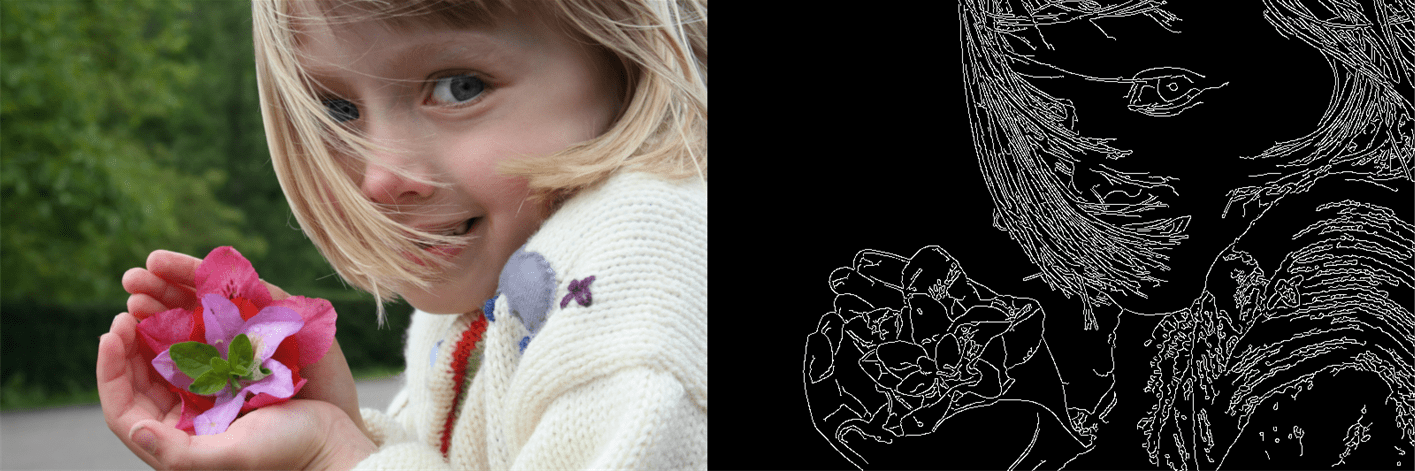So intuitively we can notice that an edge is just a group of pixels where a continues color suddenly changes for the first time. In above picture we can see that the left part of the hairs are detected thanks to the fact that the green color changes to yellow color. Same we can say the flower edges are detected because of hand color changes to pink…

Wouldn’t it be great if our NN can now detect edges?

Or even better to learn to detect type of edges by itself?

Or even more better to learn type of edges that will help to improve the model by predicting with higher accuracy?

### Data Representation

Images are usually represented as a matrix of pixels where each entry have three values from R(red),G(green), B(blue)(RGB System). So more specifically is a 3 dimensional array image[i][j][k] where i , j represents a pixel position(ixj size can be 1280×800 depending on image resolution) and image[i][j] is Red value, image[i][j] Green value, image[i][j] Blue value.

### Vertical Edge Detector

Lets see how vertical edge can be detected step by step and than we would see why this works and give a high level intuition behind it.

Lets suppose we have a simple image like half white and half black (ignoring the contour)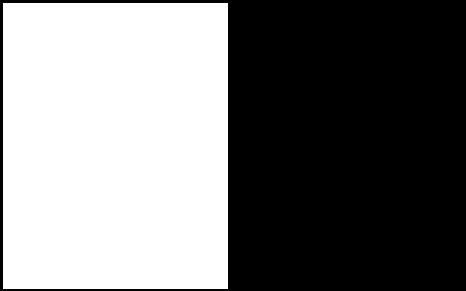Lets suppose for the sake of a better visualization that the image resolution is really small like 8×6 so 48 pixels. Additionally we will suppose that the image is represented in the Gray Scale. In contrast to RGB where we have a three dimension matrix like image[i][j][k] (where i,j are pixel position and k(0,1,2) indexes for Red,Green,Blue color values) in the Gray Scale we have only a two dimensional matrix like image[i][j] where i,j is still the pixel position but we have a single value range from 0-255 where 0 is black and 255 is white.

Our simple image will look like below to a computer: |255|255|255|10|10|10| |255|255|255|10|10|10|

The values there are perfect white 255(for the left side) and almost perfect black 10 (for the right side). The reason we did not put 0 as perfect black is just because a non zero value is better helping to explain the topic.

We will introduce another small matrix 3×3 called a filter(a vertical one). |1|0|-1| |1|0|-1|

Now lets introduce a new operation ***** called convolution as below:Convolution is fairly simple operation once you see it in action: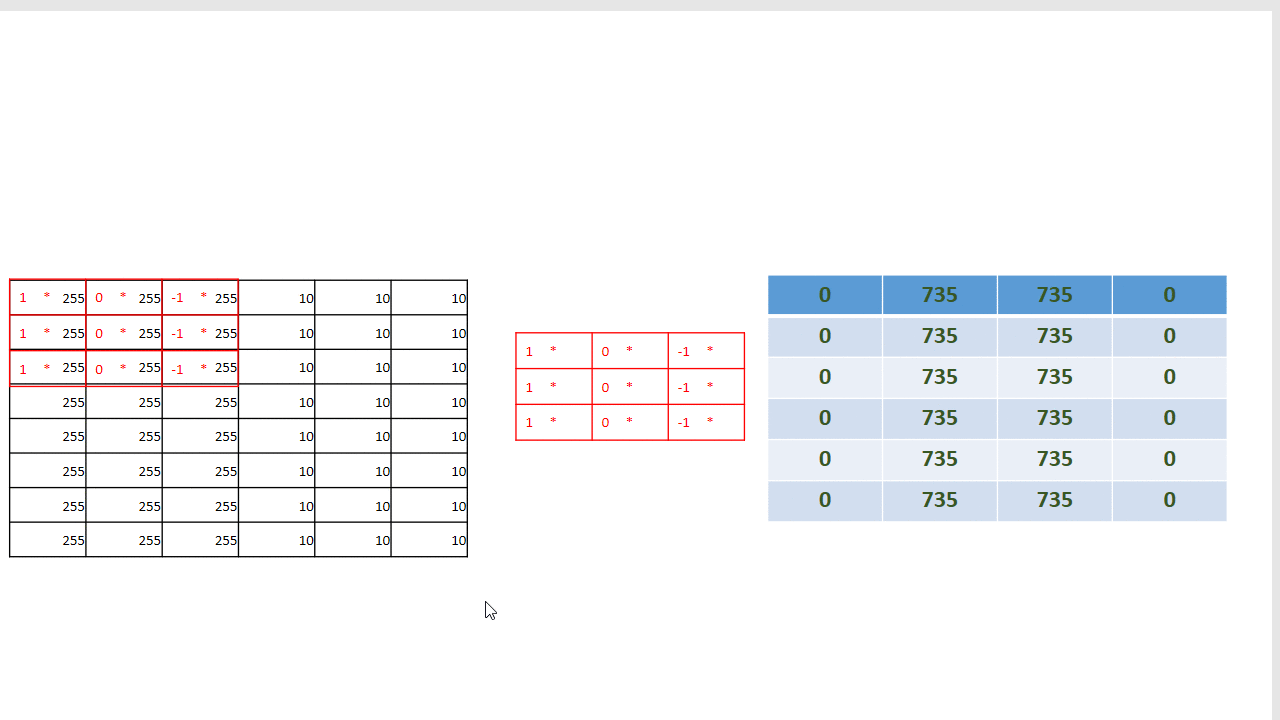As you already notice by now we are just sliding the filter one position horizontally and vertically until the end. Each time we multiply the filters matrix elements with the selected area(sub-matrix) on the image matrix and than add all together. So for position (0,0) we do:

(1 * 255 + 0 * 255 + -1 * 255 )+ (1 * 255 + 0 * 255 + -1 * 255) +( 1 * 255 + 0 * 255 + -1 * 255) = 0

This new operation produces as we can see the below new matrix: |0|735|735|0| |0|735|735|0|

One of the first observation is that this new matrix dimension have shrink from 8×6 to 6×4 pixels(more on this later).

And most importantly we can see that in the middle matrix has high non zero values while on two sides just zeros. Recalling the fact that we wanted to detect vertical edges in a black and white image lets see if this helps. If we transform this matrix back into an image while keeping in mind 0 is black and 255 or greater is white will get something like this:The image now has a white area right on the middle ,exactly where we were looking for the edge on simple black and white image. If you are wondering about the fact that the white area indicating the edge is bigger than sides, well this is due to our small 8×6 pixels example. If we were going to pick up wider image like 8×20 pixels the white are will be much smaller in comparison to the sides.

There is an simple implementation of EdgeDetection if you would like to  try it on your own.Executed on a bigger black and white image of 466×291 pixels the results would look like below:The edge now is clearly visible and also much smaller than the sides.

### Horizontal Edge Detector

In the previous topic we explored the core concept behind Edge Detectors by giving an example of Vertical Edge Detector. In this section,similarly we will walk through an example for detecting Horizontal Edges.

First lets define our image example as below:Similarly for simplicity we will work with a 8×6 pixels image that would have the below matrix values representation: |255|255|255|255|255|255| |255|255|255|255|255|255| |10|10|10|10|10|10| |10|10|10|10|10|10|

Same as previous example 255 is representing white and 10 is representing black(0 will be the darkest black but for sake of explanation we keep some value rather than 0).

Now is time to define the filter. This time we will use a slightly different filter which is just a flipped 90oright vertical filter (horizontal filter): |1|1|1| |0|0|0| |-1|-1|-1|

If we apply the convolution like explained in previous topic we will get the below matrix values for the convolution matrix: |0|0|0|0| |0|0|0|0| |735|735|735|735| |735|735|735|735| |0|0|0|0| |0|0|0|0|

Same as before the first observation is that the matrix dimension have shrink from 8×6 to 6×4 and the second observation is that in the middle we see big non zero values in comparison to zero on sides.

Recalling the fact that we wanted to detect horizontal edges in a black and white image lets see if this helps. If we transform this matrix back into an image while keeping in mind 0 is black and 255 or greater is white will get something like this:The image has a white area right into the middle, exactly where we were looking for the edge.. The edge here is quite thick because of the small resolution we have pick up 8×6 in below example the edge will much more thinner.

There is an simple implementation of EdgeDetection if you would like to  try it on your own.Executed on a bigger black and white image of 466×291 pixels the results would look like below:### Edge Detector Intuition

What is the convolution with small matrix doing that enabled us to detect edges therefore having more specialized image features?

An edge is just group of pixels where a continues color changed for the first time into some other color. Referring our simple black and white image the edge was in middle where white change to black. Also it wouldn’t be difficult to imagine edges in more complicated images , we need just to follow the colors boundaries.

Lets see one more time our two filters: |1|0|-1||1|1|1| |1|0|-1||0|0|0| |1|0|-1||-1|-1|-1|

On the left we have the vertical filter and on the right the horizontal filter

As we saw the filters were sliding first one position horizontally until we reach the last column and than slide on position vertically and again horizontally until we reach the last row and column. Like below:Each time the filter is processing a sub matrix of the same size. In this case it processes a small sub matrix of 3×3 in the big original image matrix starting at some position depending where the filter would be. Lets see what is filter doing to this sub matrix: |1 * 255|0 * 255|-1255||1 * 255|1 * 255|1 * 255| |1 * 255|0 * 255|-1256||0 * 255|0 * 255|0 * 255| |1 * 255|0 * 255|-1*257||-1 * 255|-1 *255|-1 * 255|

On the left is the vertical filter in action and on the right is the horizontal filter in action.

Mathematically what is doing is just multiplying each position and than add up all together by getting only a number in the end. So one position movement produces a number only.

Both of the fitters are ignoring the middle row by multiplying with zero. So they are both interested only on the sides , vertical filter is interested for the left and right side and horizontal filter interested for the up and down sides.

Regardless of the sides position(left , up or right down) filters can produce two states:

1. Colors are identical on both sides. So the right and left side are black(or white or other same color) or up and the down sides are  black(or white , or other same color). So no edge here since there was not any color change just a continuation of the color. The output will be 0 as one side is positive(multiplying by 1) and the other side is negative(multiplying by -1) , adding them gives 0.

2. Sides are different color. I.e the right size was white and the left is black. Similar up side was white and the down side is black. As consequence we have an edge here as the color changed from one side to the other one. The output is non zero, it can be a negative value(from black smaller values 10 to white greater values 255)  or a positive value(from white bigger values 255 to black smaller values 10) but definitely not zero so it will signal a difference between sides.

The row in the middle was left out this time but of course it will be included next time when the filter will slide one position to the right for vertical filter and one position down for the horizontal filter.

Basically what a filter is doing is just transforming the image matrix to another image matrix where every pixel now signals an edge or not. In our case in this new matrix: if the pixel is 0 it mean no edge here so we fill it with black color. On the other hand if a pixel has a value we take the absolute(-100 or 100 is the same 100)value with a max of 255(-735 or 735 is 255)indicating the presence of an edge,  draw with white since higher number means more whiter.

## Convolution on RGB Images(Examples)

So fare we only walked through simple black and white images. Now is time to explain how convolution will work with RGB colorful images.

Recalling from Data Representation section a RGB image is represented by a three dimensional matrix *image[i][j][k] where (i,j) are the positions of pixels and k index(0,1,2) of colors value for Red,Green and Blue. *Comparing to black and white sample which had only one matrix with gray scale values from 0-255(0 black, 255 white) now we have three matrices each representing values 0-255 for Red,Green,Blue like below: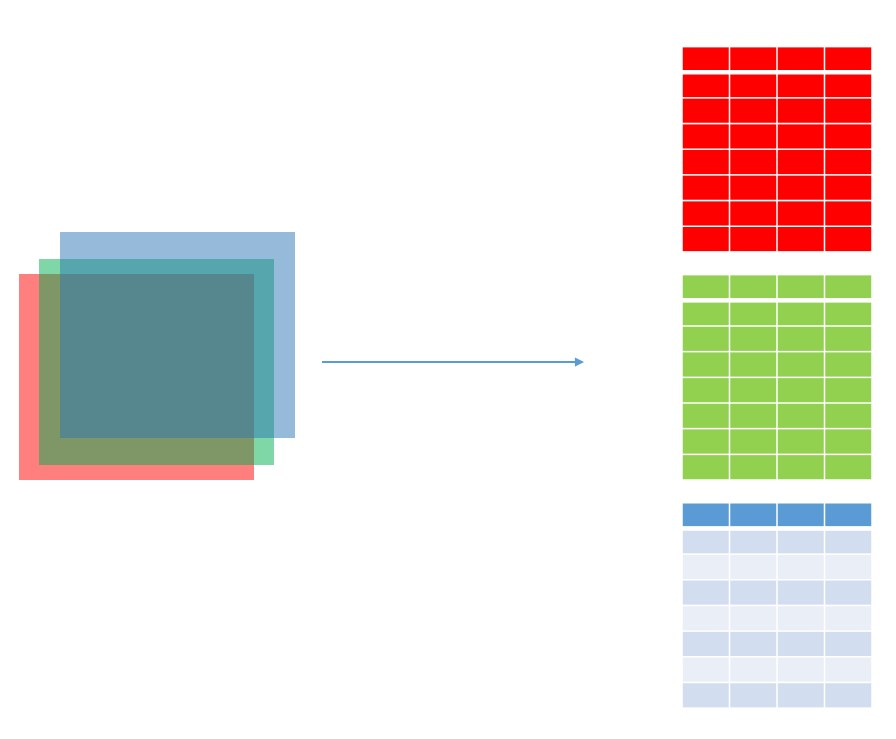The problem does not sound hard to address as now we only have three matrices instead of one. One can suggest to just apply convolution to each of them separately similarly as we did for one black and white:Well this worked quite well as we similarly to the black and white matrix have value from 0-255 for each transformed matrix. It is just that they instead of gray scale represent R,G,B respectively but a computer does not really care as long as you feed him with numbers.

Although it looks like a solution we have a one last problem to solve. As we know after the convolution the matrices pixels represent knowledge if the pixel is an edge or not. So they do not represent anymore any color information or at least not in the same format as the original matrices R,G,B. Keeping this int mind , does it really make sense to keep three of them?

Indeed keeping them separately intuitively does not seem useful beside we occupy useful memory and processing power. Maybe it will interesting research to try keeping three of them( if such research is not already existing as I am not aware of any at the moment). The solution again is pretty intuitive we just add three matrices together so in the end there is only one matrix left.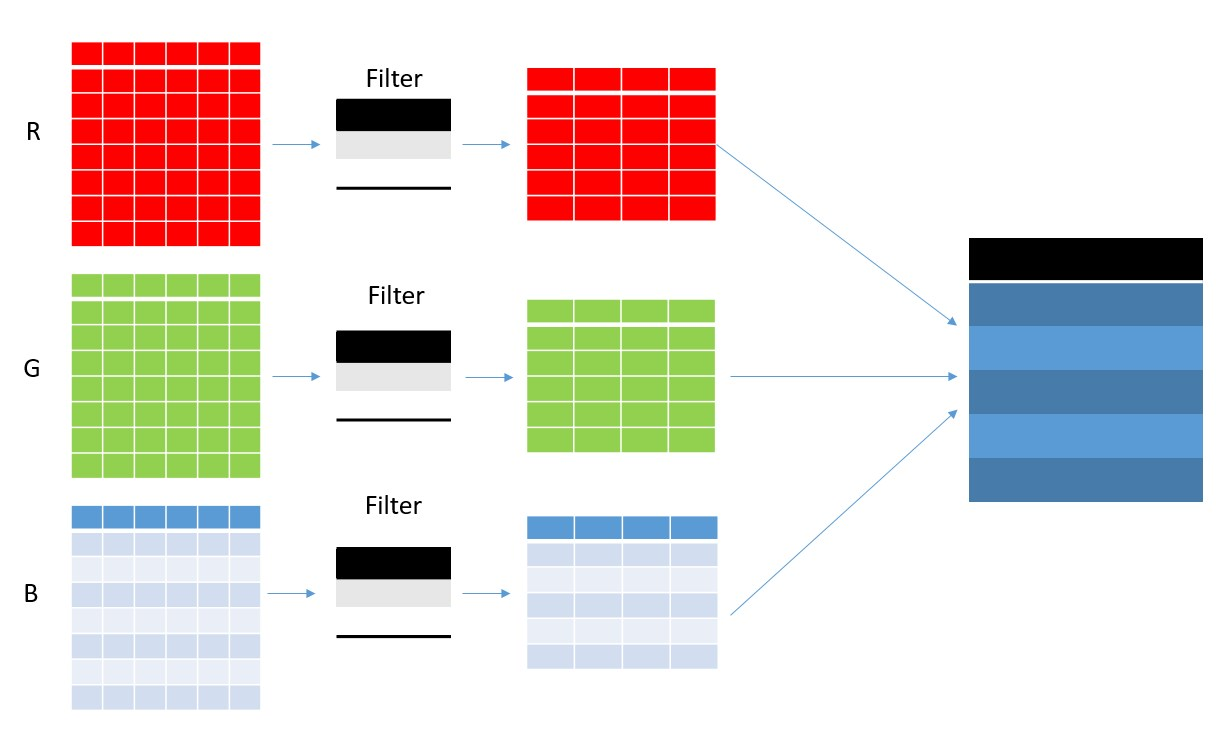As we can see convolution always will result in one single matrix regardless of the number(number of channel will be one) you had before applying it(usually 3 as RGB but it can also have more channels).

Notice that we used the same Filter for the input R,G,B matrix but in reality those filters maybe different. So the convolution of RGB(WxHx3) matrix is done with a three dimensional Filter(WxHx3 where W,H can be 5×5 but the third parameter has to be equal to input matrix 3). If the input matrix has more channels like 4,5,6 than the convolution has to have a filter with same dimension as well 4,5,6. Anyway regardless of the third dimension value(channels number) still the convolution always produces a two dimensional matrix by cutting the third dimension.

There is an simple implementation of EdgeDetection if you would like to  try it on your own. Executed on below image:it gives below results for vertical , horizontal and sobel filters:

Vertical: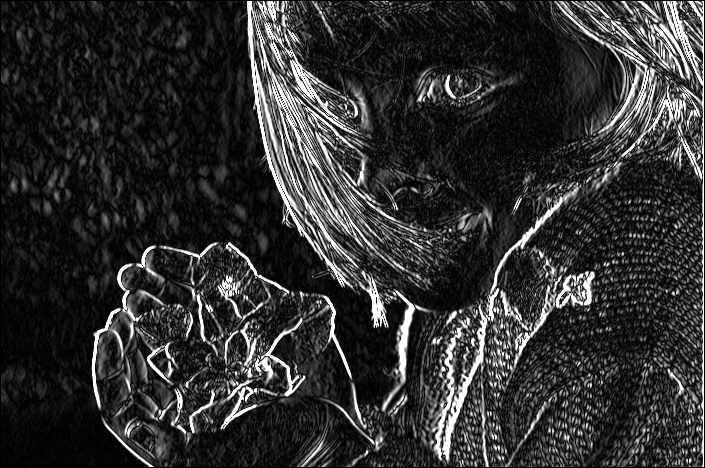Horizontal: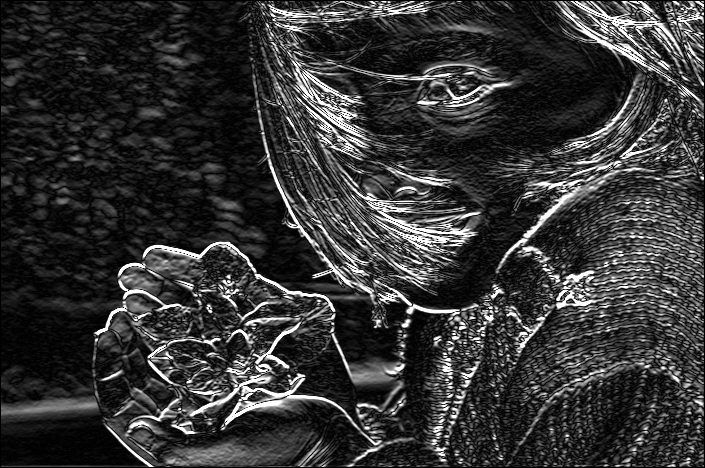Sobel:## Convolution Parameters

Already we have seen that the convolution operation:

• shrinks the matrix dimensions from 8×6 to 6×4 in our case

• results always in one matrix regardless of original number of matrices

We can of course control both of them in a way that will serve better our model. We have three ways If we would like to control the convolution matrix(CM) dimensions.

We can use padding in the case we want our CM to be equal or even greater than the original image(the third dimension is one for the black and white example). What padding does is just adding more zero value rows and columns to the original image. So in our simple black and white representation it look like below : |0|0|0|0|0|0|0|0| |0|255|255|255|10|10|10|0| |0|255|255|255|10|10|10|0| |0|0|0|0|0|0|0|0|

We changed the dimension from8×6 to 10×8 by applying 1 padding(one zero layer all around). Now if we apply a 3×3 convolution filter as explained above to this new 10×8matrix(by sliding one position horizontally and than one position vertically) we will get a CM with dimension 8×6, same as the original image. Padding more will result CM to be even greater than original image. By now you already guessed that there is a specific formula by which original image dimension is related to the CM. The formula is fairly easy as below: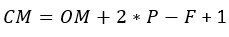where CM is convolutional matrix dimension(column or row) , OM is original matrix dimension (column or row) , P stands for padding number and F is the filter dimensions(column or row).

So if we would like to have the CM matrix same dimension as OM than we need just to solve the above equation to be equal to original matrix dimension: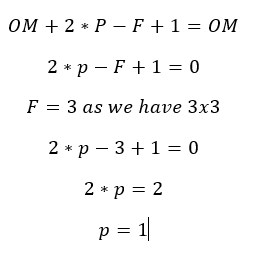We found that padding by one will give us the same dimension as original matrix after applying the 3×3 convolution.

Padding by one make the 8×6matrix evolve to 10×8.Lets try to apply convolution now:

CMrow =OMrow + 2 * P- Frow +1 =  8 +(1 * 2) – 3 +1 =  8

CMcol =OMcol + 2 * P – Fcol +1 =  6 + (1 * 2) – 3 +1 =  6

#### Stride

We can use stridingin the case we would like to shrink our CM(the third dimension is always one) even more as by default it always shrinks a bit. Until this point we always slide the filter on position , what if we can slide 2,3,4? Sliding the filter with greater factor will result the CM to have smaller dimensions as we simply check less elements on the original matrix. The reason we might want to do that is maybe to save some computation and memory so to say maybe this is not impacting our model in a bad way but instead it speeds the learning phase. Of course again there is formula: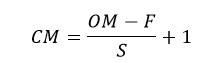where CM is convolutional matrix dimension(column or row) , OM is original matrix dimension (column or row) , S stands for striding number of positions and F is the filter dimensions(column or row).

So basically S reduces CM dimension by a great factor, in the minimum case is S=2 which means the dimension are divided by two.

#### Multiple FIlters

Above we saw how to control CM dimensions on rows and columns but still we always had the third dimension set to one(convolution always produces one two dimensional matrix). This is because as we saw convolution will add upall CM matrices into a one CM matrix and this is happening regardless of original matrix had a third dimension(RGB). What we can do here is add more filters and apply convolution to the original matrix for each of this filters. By doing so we end up with multiple CM’s for each filter. So to say if we add 3 filters(each three dimensional or 3 channels as the original matrix) we would end up with 3 CM’s same as original RGB matrix. Below is how this may look like:#### Putting all together

So in three above section we learned how to control dimension rows and columns through padding and striding and the number of the third dimension(channel) by adding more filters.

We can of course combine both padding and stridingin one final formula which gives us the flexibility to control matrix dimensions: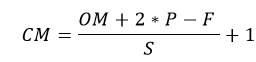where CM is convolutional matrix dimension(column or row) , OM is original matrix dimension (column or row) , P stands for padding number, S stands for striding number of positions and F is the filter dimensions(column or row).

Was not mention above but of course we can shrink the size of CM by also increasing the size of the Filter itself(F). Till not we saw only a 3×3 filter but for sure it may change and from formula we can see that the greater the filter dimensions smaller the CM dimension(keeping other parameters constant).

The third dimension(channel) is easy to think about as the number of filters affects directly the size of CM channels or third dimension.

It is possible to produce the same size as the input after applying a convolution ,we just need to solve the above equation to equal to the input size. Usually when we want the same size matrix also after the convolution is applied the operation is called : ‘Same Convolution‘.

## Pooling Layers

Pooling layers are another type of filter that usually used for reducing matrix dimensions therefore speed up the computations. Most of the times is used Max Poolingbut maybe also rarely Average Pooling.

### Max Pooling

Max Pooling(MP) is very similar to filters for edge detection. MP instead of multiplying and than adding all pixels of the selected sub matrix they just produce as output the maximum value of selected pixels. In the same way as filters they slide one ore more positions horizontally and vertically depending on parameters. So they share exactly  the same formula as filters for controlling the output dimensions.IM is input matrix dimension (column or row) , P stands for padding number(usually 0), S stands for striding number of positions(usually 2) and F is the max pooling dimensions(column or row, usually 2×2).

One thing to notice about Max Pooling is that they leave the third dimension untouched(same value as it was). We saw that convolution filters cut the third dimension by always producing two dimensional matrix. While Max Pooling are used only to reduce heightXwidth and leaving third dimension unchanged. This is done by applying the same Max Pooling Filter as much times as the third dimension value was on the original Matrix.( as we saw at Multiple Filters section).

There is not really much to say about Max Pooling as they share every dynamics with filters. Is hard to say what is the intuition behind Max Pooling but usually they work well to reduce dimension therefore speed up computation, reduce overfitting  and improve also the model accuracy.

### Average Pooling

As the name suggest this Pooling Layer instead of doing the max or multiply and add they calculate the average of selected pixels.In practice is found very rarely as more often a Max Pooling will perform better.

## Learn with Convolution Neural Network

Now is time to put all pieces together in building a model which can actually learn and than help us predict and solve problems. The part that doesn’t change here is the Neural Network (NN)we already saw. We only add what is seen so fare before NN start learning, so to say NN now will learn in a more image specialized features processed by Convolutional Technics.

Anyway there is a final trick we need to discover to make CNN even more powerful. So fare we have seen only a limited number of filters : Vertical Edge Filter, Horizontal and Sobel(by example only).

Why can’t we just let the NN figure out what type of filters(edge detectors) are better for the model? Maybe NN can find out better filters than Vertical,Horizontal or Sobel. The final trick consist of parameterizing the filters so instead of hard coding the types they can be discovered by NN. In the same time lets add also many parameterized filters to the model. So it may look as below: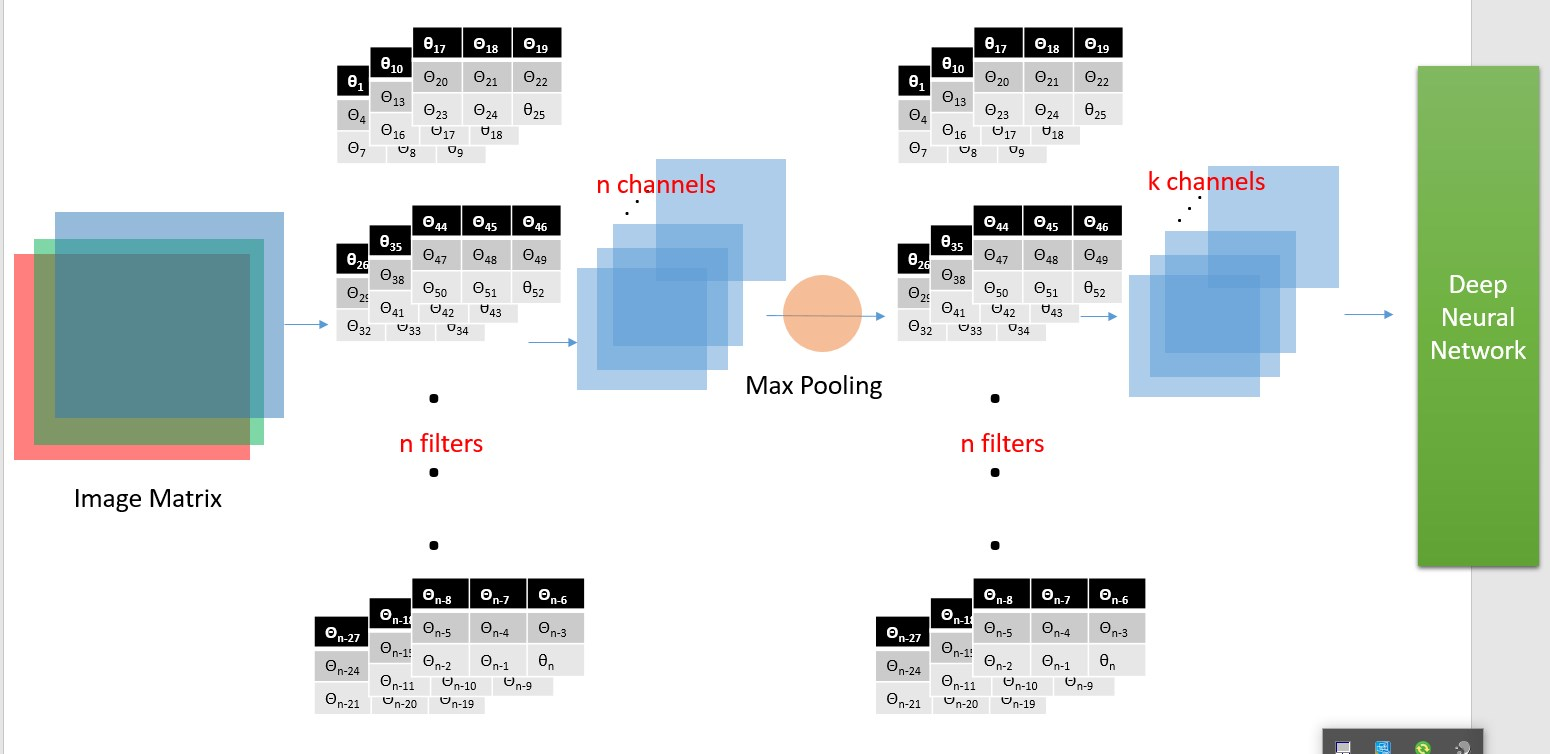Filter Θ parameters introduced by filters are identical to NN Θ , they are learned by the network with the help of back propagation as explained at previous post. Although there is a small difference with NN parameters, filters parameters are smaller in number so they will not slow down back prop and in the same time offer great features for our model.

E.x if we add 16 filters with 5x5x3 dimension and another layer of 32 filters of 3×3x3 dimension we end up with 2064 parameters. If we take the same example but with neurons :

Input size 28×28 pixels so 784 ,  two hidden layers (one with 16 neurons and the other with 32) and 10 outputs in total they produce : 784 * 16 * 32 * 10 = 4.014.080 parameters to learn. So the neurons parameters numbers are much larger because they depend on :  fully connected inputs ,hidden layers and outputs. While filters parameters remain constant therefore not slowing down that much.Anyway Still convolutional networks take a long time to train on simple computers because of large multiplication operation number comparing to Neural Networks without convolution layers.

On the other hand Pooling Layers do not have any parameters they just serve as a transformer or better as a dimension reducer of the input matrix.

## Application

### Data Source

Data used for building the application were taken from this web site :

MNIST database has 60.000 of training data and 10.000 of test data.  The data contain black white hand written digit images of 28X28 pixels. Each pixel contains a number from 0-255 showing the gray scale, 0 while and 255 black. Data used here are the same as previous post so for more details how the data are organised please have a quick look here.

### Network Configuration

Same as previous post we usetwo hidden layers 128 and 64 neurons and 10 outputs(0-9 digits). But of course there is crucial difference in front of two hidden layers(before 128 neurons) we apply several convolution layers and max pooling like below:Applying the formula we explored at “Putting All Together Section” we get the following dimensions:

First we apply a 20 convolution operations with size 5×5(S=1,P=0) so that gives us: 28-5/1 +1=24 so 24×24 and since we have 20 convolutions we have 24x24x20

Second we apply max pooling with 2×2 and s=2 so this gives us:                           24-2/2 +1= 11 so 12x12x20.

Again we apply 50 convolution(5×5 S=1,P=0) and max pooling (2×2 s=2,P=0) and in the end we have 4x4x50(800) image size. We feed the new image to a neural network with 4x4x50(800) as input size, two hidden layers 128,64 and one output 10(0-9 digits). Lets see below how the code will look like.

### Code

In previous post we used Spark MLib train a Simple Neural Network and predict hand writing digits. While in this post we used Deeplearning4j framework to train a deep convolutional network. The reason behind that is that SPARK MLib at the moment do not offer convolution layers to a Neural Network. Please find below the network configuration matching above topology(for more details find class code here):

### Application and Results

Training the network this time took approx 1.5 hours and required almost 10GB of RAM memory. As above code shows the network was trained 20 times with a batch size of 64. The accuracy was quite respectable 99.2%in comparison to 97% previously without using Convolution.

The model was still improving and maybe running 20 more times would improve the accuracy even more but running convolution neural networks takes a lot of resources and time. For this type of applications GPU will greatly help in training bigger neural networks and much faster. Feel free to try more deep network or this topology with more epochs. There also already trained networks MNIST DataSet like the well known LeeNet-5. In the source you already can find an implementation of LeeNet-5 which when I run it offered 99% accuracy in 15 iterations also quite fast training(maybe better for prototyping).

### Run Application

Application can be downloaded and executed without any knowledge of java beside JAVA has to be installed on your computer. Feel to try it with choosing different options like:

• training data size default to 30.000 and test data size default to 10.000

• number of neurons and layers, not yet added(contributions appreciated)

Application already loads a default training executed before hand with accuracy 99.2% tested in 10.000 of test data and trained with 60.000 images.

!!Please try to draw in the center as much as possible as the application do not use centering or crop as the data used for training.

We can run the from source by simply executing the RUN class or if you do not fill to open it with IDE just run mvn clean install exec:java..

Application was build using Swing as GUI and DeepLearning4J for the executing the run.bat would show the below GUI: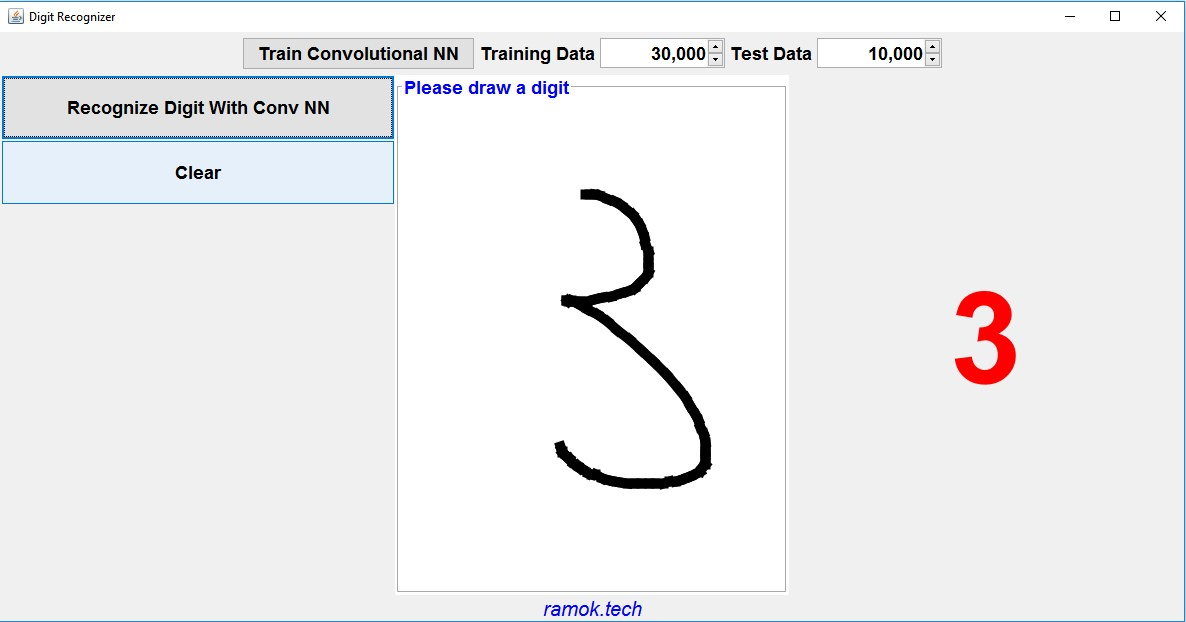Found useful , feel free to share